# Implicit Differentiation Lesson 6 4 Tangent to a

• Slides: 11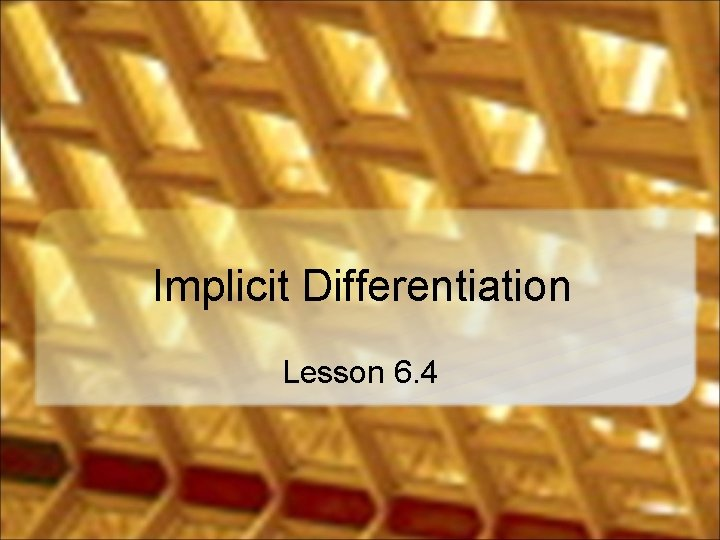Implicit Differentiation Lesson 6. 4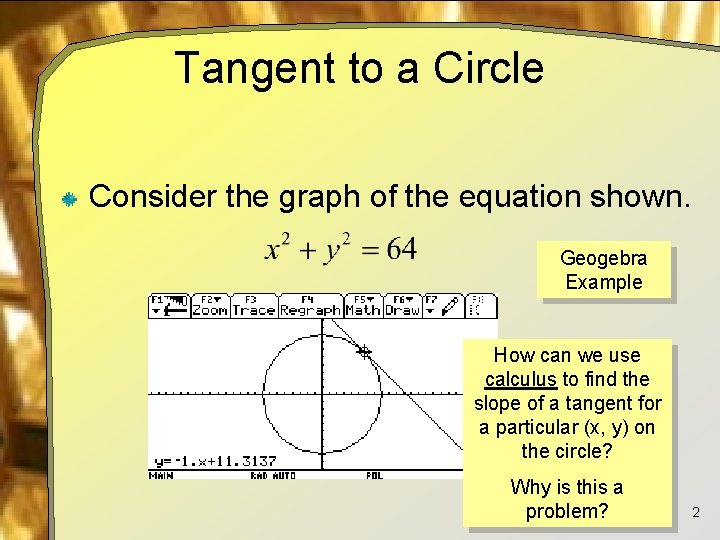Tangent to a Circle Consider the graph of the equation shown. Geogebra Example How can we use calculus to find the slope of a tangent for a particular (x, y) on the circle? Why is this a problem? 2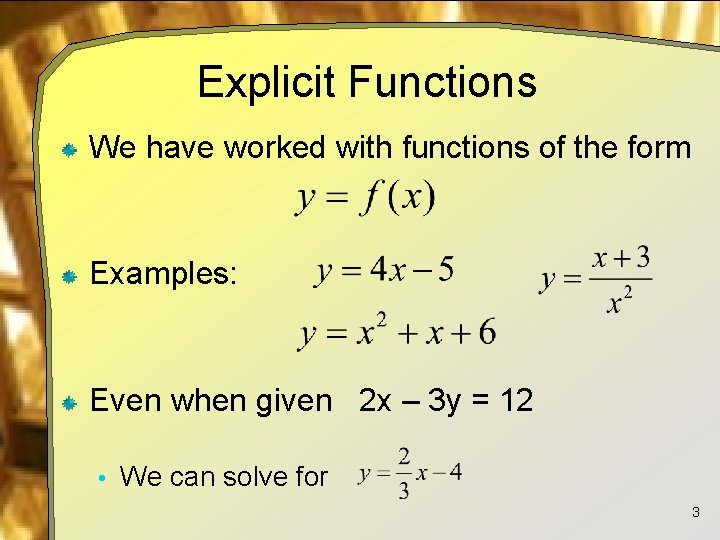Explicit Functions We have worked with functions of the form Examples: Even when given 2 x – 3 y = 12 • We can solve for 3Implicit Functions Some functions cannot be readily solved for y. For these we say y is given implicitly in terms of x We will use implicit differentiation to find 4Implicit Differentiation Given 4 xy – 6 y 2 = 10 • We differentiate with respect to x on both sides of the equation Use product rule and chain rule Each time an expression has a y in it, we use the chain rule Use chain rule 5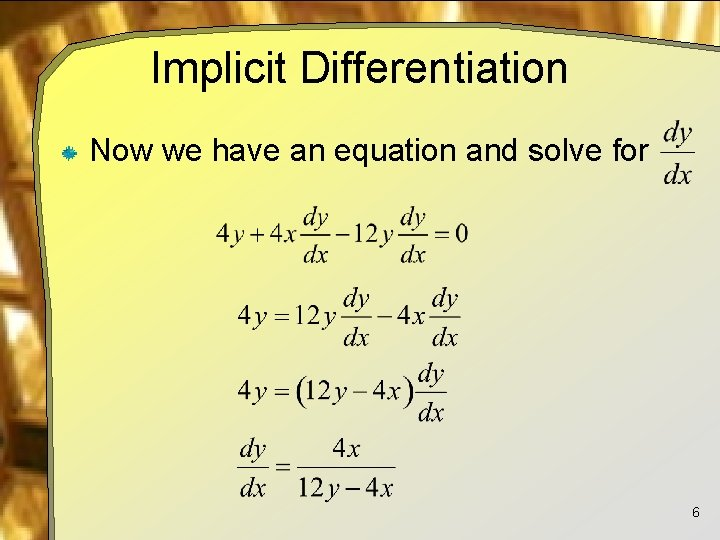Implicit Differentiation Now we have an equation and solve for 6We Better Try This Again Find dy/dx for following 7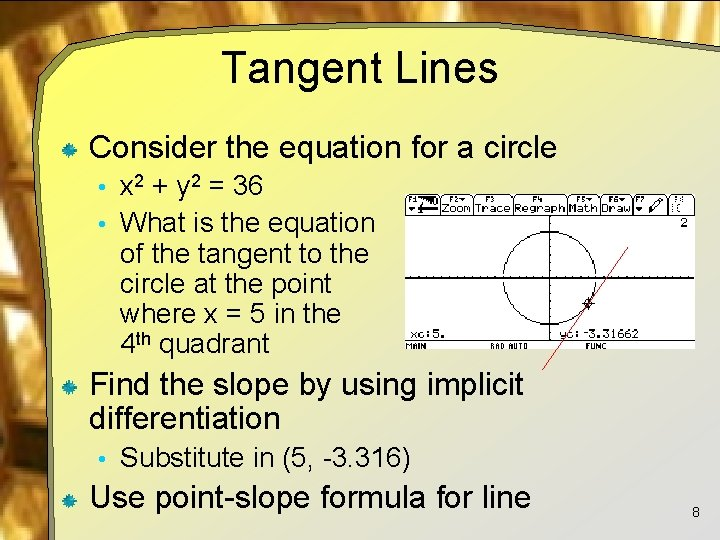Tangent Lines Consider the equation for a circle • x 2 + y 2 = 36 • What is the equation of the tangent to the circle at the point where x = 5 in the 4 th quadrant Find the slope by using implicit differentiation • Substitute in (5, -3. 316) Use point-slope formula for line 8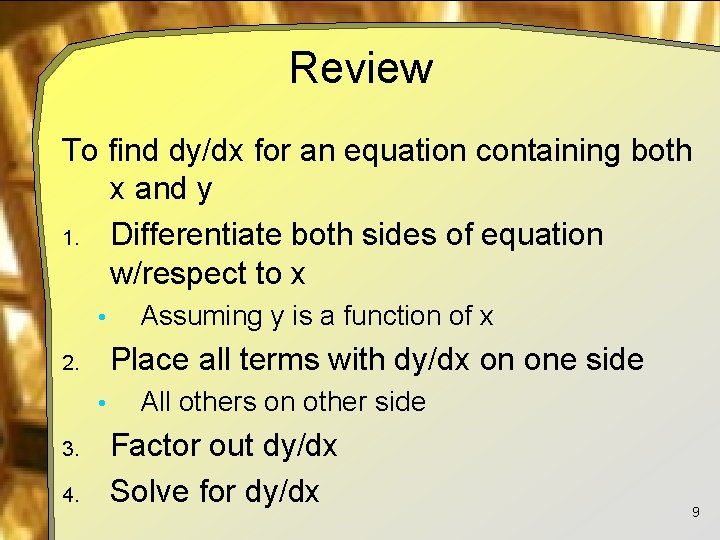Review To find dy/dx for an equation containing both x and y 1. Differentiate both sides of equation w/respect to x • Place all terms with dy/dx on one side 2. • 3. 4. Assuming y is a function of x All others on other side Factor out dy/dx Solve for dy/dx 9Implicit Differentiation on the TI Calculator We can declare a function which will do implicit differentiation: Note, this cannot be an equation, only an expression Usage: 10Assignment Lesson 6. 4 Page 401 Exercises 1 – 21 odd, 43 11